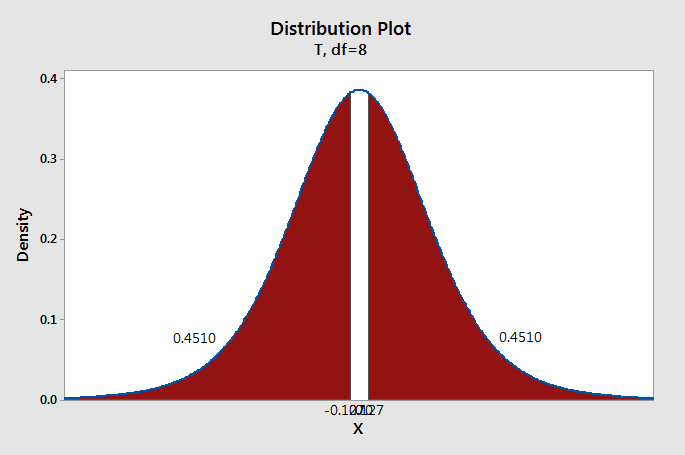# 8.3.2 - Hypothesis Testing

8.3.2 - Hypothesis Testing

Below are the procedures for conducting a hypothesis test for two paired means. This is often referred to as a "paired means $$t$$ test," "dependent means $$t$$ test," or "matched pairs $$t$$ test."

1. Check any necessary assumptions and write null and alternative hypotheses.

Data must be paired. The difference between the two groups must be normally distributed in the population or the sample size must be at least 30.

The possible combinations of null and alternative hypotheses are:

Research Question Is the mean difference different from 0? Is the mean difference greater than 0? Is the mean difference less than 0?
Null Hypothesis, $$H_{0}$$ $$\mu_d = 0$$ $$\mu_d = 0$$ $$\mu_d = 0$$
Alternative Hypothesis, $$H_{a}$$ $$\mu_d \neq 0$$ $$\mu_d > 0$$ $$\mu_d < 0$$
Type of Hypothesis Test Two-tailed, non-directional Right-tailed, directional Left-tailed, directional

Where $$\mu_d$$ is the hypothesized difference in the population.

2. Calculate an appropriate test statistic.

The calculation of the test statistic for dependent samples is similar to the calculation you performed earlier in this lesson for a single sample mean. In this formula, $$\overline{x}_d$$ is used in place of $$\overline{x}$$ and $$s_d$$ is used in place of $$s$$:

Test Statistic for Dependent Means

$$t=\frac{\bar{x}_d-\mu_0}{\dfrac{s_d}{\sqrt{n}}}$$

$$\overline{x}_d$$ = observed sample mean difference
$$\mu_0$$ = mean difference specified in the null hypothesis
$$s_d$$ = standard deviation of the differences
$$n$$ = sample size (i.e., number of unique individuals)

Observed Sample Mean Difference
$$\overline{x}_d=\dfrac{\Sigma{x}_d}{n}$$
$$x_d$$ = observed difference
Standard Deviation of the Differences
$$s_d=\sqrt{\dfrac{\sum (x_d-\overline{x}_d)^{2}}{n-1}}$$
3. Determine the p value associated with the test statistic.

When testing hypotheses about a mean difference, a $$t$$ distribution is used to find the $$p$$ value. The degrees of freedom are equal to $$n-1$$ where $$n$$ is the number of pairs.

4. Decide between the null and alternative hypotheses.

If $$p \leq \alpha$$ reject the null hypothesis. If $$p>\alpha$$ fail to reject the null hypothesis.

5. State a "real world" conclusion.

Based on your decision in Step 4, write a conclusion in terms of the original research question.

# 8.3.2.1 - Example: Quiz Scores

8.3.2.1 - Example: Quiz Scores

Below is an example of conducting a paired means $$t$$ test by hand using raw data. Next, you will learn how this can be conducted most efficiently in Minitab.

Research question: Are scores on two quizzes different?

Data were collected from 9 students and a paired means $$t$$ test was performed using hand calculations:

Student ID Quiz 1 Quiz 2
001 98 94
002 100 98
003 95 98
004 90 88
005 90 89
006 92 91
007 80 84
008 78 80
009 88 88
Step 1: Check assumptions and write hypotheses

There are two assumptions: (1) data are paired and (2) distribution of differences is normally distribution in the population or the sample size is at least 30. The data are paired because for each student we have a quiz 1 and a quiz 2 score. We do not know if the differences are normally distributed in the population and the sample size is small, but in the video above we created a histogram of the differences and found that the sample was approximately normally distributed, so this assumption has been met and we can perform a paired means $$t$$ test.

Given $$\mu_d = \mu_1 - \mu_2$$, our hypotheses are:
$$H_0: \mu_d = 0$$
$$H_a: \mu_d \ne 0$$

Step 2: Calculate test statistic
Test Statistic for Dependent Means

$$t=\frac{\bar{x}_d-\mu_0}{\frac{s_d}{\sqrt{n}}}$$

$$\overline{x}_d$$ = observed sample mean difference
$$\mu_0$$ = mean difference specified in the null hypothesis
$$s_d$$ = standard deviation of the differences
$$n$$ = sample size (i.e., number of unique individuals)

Student ID Quiz 1 Quiz 2 Difference ($$X_d$$) $$X_d - \overline{X}_d$$ $$(X_d - \overline{X}_d)^2$$
001 98 94 4 3.889 15.123
002 100 98 2 1.889 3.568
003 95 98 -3 -3.111 9.679
004 90 88 2 1.889 3.568
005 90 89 1 0.889 0.790
006 92 91 1 0.889 0.790
007 80 84 -4 -4.111 16.901
008 78 80 -2 -2.111 4.457
009 88 88 0 -0.111 0.012

Mean of the differences: $$\overline{X}_d=\frac{\Sigma{X}_d}{n}=\frac{1}{9}$$

For a review of computing standard deviation, see Lesson 2.

Sum of squares: $$\Sigma (X_d - \overline{X}_d)^2 = 54.889$$

Standard deviation of the differences: $$s_d=\sqrt{\frac{\sum (X_d-\overline{X}_d)^{2}}{n-1}} = \sqrt{\frac{54.889}{9-1}}=2.619$$

Test statistic: $$t=\frac{\overline{X}_d- \mu_0}{\frac{s_d}{\sqrt{n}}}=\frac{\frac{1}{9}}{\frac{2.619}{\sqrt{9}}}=0.127$$

$$df=n-1=9-1=8$$

Step 3: Determine p-value

We can construct a $$t$$ distribution with 8 degrees of freedom and determine what proportion of the curve falls beyond a $$t$$ score of 0.127. This is a two-tailed test, so we need to take into account both the left and right sides of the curve.$$p=0.4510+0.4510=0.9020$$

Step 4: Make a decision

We will compare our $$p$$-value from step 3 to a standard alpha level of 0.05.

Because $$p>\alpha$$, we fail to reject the null hypothesis.

Step 5: State conclusion

There is not sufficient evidence to state that scores on the two quizzes are different.

Note! The following video uses Minitab Express not Minitab to find the p-value

  Link ↥ Has Tooltip/Popover Toggleable Visibility